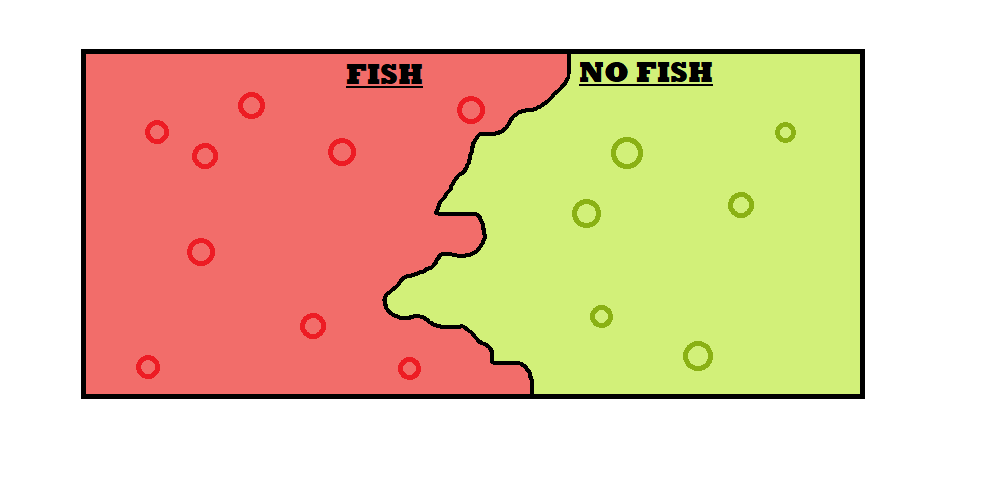Trusted answers to developer questions
Trusted Answers to Developer Questions

Related Tags

knn
python
machine learning
communitycreator

# What is the KNN algorithm?

Irzum Jafri

Grokking Modern System Design Interview for Engineers & Managers

Ace your System Design Interview and take your career to the next level. Learn to handle the design of applications like Netflix, Quora, Facebook, Uber, and many more in a 45-min interview. Learn the RESHADED framework for architecting web-scale applications by determining requirements, constraints, and assumptions before diving into a step-by-step design process.

KNN stands for K- Nearest Neighbors. It is the simple supervised machine learning algorithm which is extensively used to solve classification and regression problems. It has wide applications in the field of machine learning.

### Supervised machine learning

The supervised machine learning algorithm is one that depends on the labeled input, then learns from that input dataset, and creates a function that produces an output (prediction) of unlabeled data based on the learning.

#### Example

Let’s imagine a computer as a small child. We want it to learn what a fish is. To make a child learn about the fish, we show him the fish, and we tell him, “This is a fish.” Sometimes, the child points at a creature and asks if it is a fish, and if the creature is not fish, we tell him, “No, it’s not a fish.”

Similarly, we provide a computer with a labeled dataset in which input and output are provided. The algorithm learns from that dataset, and after that, we test the algorithm by providing only the input. Based on the previous learning, the algorithm predicts its output.

### KNN

In the KNN algorithm, we train the model using a dataset. The algorithm classifies the dataset into different categories. Like in the previous example, some pictures are fish and some pictures are not fish.KNN captures the idea of similarity. It finds the distance between the points in the graph and the test data for which output is needed, and based on k nearest neighbors, which can be chosen, it tells the output of the test data.

### Distance methods

One of the basic methods of finding the distance is using Euclidean or straight line distance. We can find the Euclidean distance between the two using the following code.

#### Code

def euclidean_product(x,y):    dist = np.linalg.norm(x - y)    return dist
def euclidean_distance(train, test):    train = train.to_numpy()    test = test.to_numpy()    ED =[]    for x in  test:        ED_row = []        for y in train:            ED_row.append(euclidean_product(x,y))        ED.append(ED_row)    return np.array(ED)

### The algorithm

The KNN algorithm is implemented in the following steps:

1. Load the dataset.
2. Initialize the value of k.
3. Calculate the distances of the data points with the vale being compared and make a list.
4. Sort the list in ascending order and only keep the lowest k entries.
5. Get the labels of these selected k entries.
6. Return the mean, or average value, of these entries if regression is being done and the mode, the most repeated value, if it is classification.

### Implementation

To implement KNN, we will be using sklearn library. It provides us with predefined functions to implement the KNN algorithm.

The code is given below:

from sklearn.neighbors import DistanceMetricfrom sklearn.neighbors import KNeighborsClassifierfrom sklearn.metrics.pairwise import manhattan_distancesfrom sklearn.metrics.pairwise import euclidean_distancesfrom sklearn import metricsfrom sklearn.metrics import classification_report, confusion_matrixclassifier = KNeighborsClassifier(n_neighbors=k+1,metric='euclidean')classifier.fit(train_X, train_Y)PL = classifier.predict(test_X)
Acc_score = (metrics.accuracy_score(test_Y,PL))*100f1_scores = (metrics.f1_score(test_Y, PL, average='macro'))

RELATED TAGS

knn
python
machine learning
communitycreator

CONTRIBUTOR

Irzum Jafri

Grokking Modern System Design Interview for Engineers & Managers

Ace your System Design Interview and take your career to the next level. Learn to handle the design of applications like Netflix, Quora, Facebook, Uber, and many more in a 45-min interview. Learn the RESHADED framework for architecting web-scale applications by determining requirements, constraints, and assumptions before diving into a step-by-step design process.

Keep Exploring

Learn in-demand tech skills in half the time